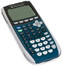Practice with Standard Deviation Terms of Use    Contact Person: Donna Roberts1.  Your task is to survey all of the students in your mathematics class to determine the number of pets owned by the students' families. A statistical analysis will be prepared on the class data, but no generalizations will be made to larger groups of students.Will the collected data be a population data set or a sample data set?
 Choose: population sample

 2.  Data: 22, 24, 15, 18, 27, 16, 23, 20, 19, 26(a) Find the mean.
 Choose: 18 21 22 24

(b)
Find the sample standard deviation.

 Choose: 3.87 3.94 4.08 4.12

(c)
Find the population standard deviation.

 Choose: 3.87 3.94 4.08 4.12

3.  Abby collected data on the number of students enrolled in freshman math classes in her high school.

Which statement is true regarding the range of her data?

 Size of Class Frequency 20 1 22 4 28 2
 Choose: range = mean range > mean range < mean range < standard deviation

4.  Kevin's chemistry scores are 86, 88, 87, 86 and 88.
Josie's chemistry scores are 84, 72, 96, 71 and 80. Which statement is true regarding the standard deviations of these scores?Choose:
 The standard deviations for both sets of scores are the same. The standard deviation for Kevin's scores is greater than the standard deviation for Josie's scores. The standard deviation for Kevin's scores is less than the standard deviation for Josie's scores. The standard deviation cannot be computed on only five scores.

5.  Data: 10, 12, 12, 13, 17, 19, 20
What is the difference between the sample standard deviation and the interquartile range for this data, to nearest hundredth?

 Choose: 3.1 3.39 0.39 4.016.  Maria and Ramon are competing in a downhill ski competition. Maria notices that the mean of her time scores is identical to that of Ramon. She also notices that the standard deviation of Ramon's time scores is 12.3 seconds, while her standard deviation is 2.6 seconds. Which statement must be true?Choose:
 The median for Maria's scores must be lower than the median for Ramon's scores. Maria's scores are more consistent than Ramon's scores. Ramon's scores are more consistent than Maria's scores. The median for Ramon's scores must be lower than the median for Maria's scores.

7.  Dylan's dart game scores are:
480, 320, 420, 380, 460, 400.
Which set of values represents the standard deviation of the scores, followed by the number of scores that are within one standard deviation of the mean?
(population standard deviation rounded to nearest tenth)Choose: 57.6; 3 52.6; 4 57.6; 4 52.6; 3

8.  The rainfall in a small town is recorded daily for two weeks, in inches: 1.2, 2.3, 0.8, 2.3, 1.0, 3.1, 4.2, 1.4, 2.1, 0.4, 1.3, 2.0, 1.8, 3.2.

What are the mean and the sample standard deviation for this data set (to the nearest hundredths)?

Choose:
 mean = 1.94; standard deviation = 1.04 mean = 1.93; standard deviation = 1.01 mean = 1.94; standard deviation = 1.01 mean = 1.93; standard deviation = 1.04